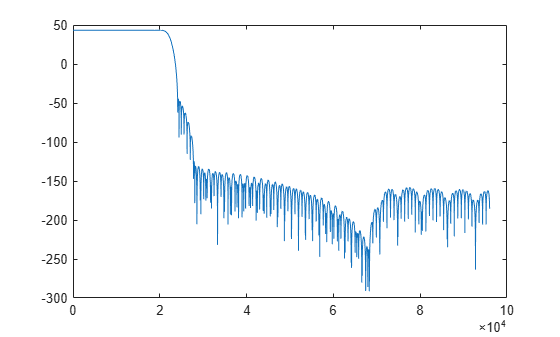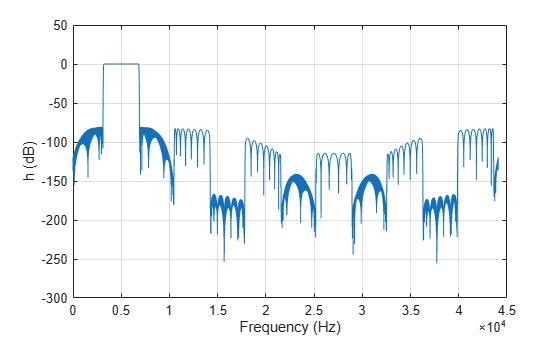freqz

Frequency response of the multirate multistage filter

Description

example

[h,f] = freqz(sysobj,n,range) returns the complex frequency response, h, of the multirate multistage filter System object™ and the frequency vector f at which h is computed. n is the number of frequency points, and range is the frequency range over which the response is computed.

For the sample rate converter object, the sample rate is the larger of InputSampleRate and OutputSampleRate.

example

[h,f] = freqz(sysobj,f) returns the complex frequency response h computed at the frequency points specified by vector f. The input vector f is in Hz.

Examples

collapse all

Create a multistage sample rate converter with default properties, corresponding to the combined three filter stages used to convert from 192 kHz to 44.1 kHz. Compute and display the frequency response.

src = dsp.SampleRateConverter;
[H,f] = freqz(src);
plot(f,20*log10(abs(H)))Compute and display the frequency response over the range between 20 Hz and 44.1 kHz.

f = 20:10:44.1e3;
[H,f] = freqz(src,f);
plot(f,20*log10(abs(H)))Compute the complex frequency response of a complex bandpass decimator using the freqz function.

Create a dsp.ComplexBandpassDecimator object. Set the DecimationFactor to 12, the CenterFrequency to 5000 Hz, and the SampleRate to 44100 Hz. Compute and display the frequency response.

cbp = dsp.ComplexBandpassDecimator(12,5000,44100);
[h,f] = freqz(cbp);
plot(f,20*log10(abs(h)))
grid on
xlabel('Frequency (Hz)')
ylabel('h (dB)')Input Arguments

collapse all

Filter System object, specified as a dsp.ComplexBandpassDecimator or a dsp.SampleRateConverter System object.

Number of frequency points for response evaluation, specified as a positive integer scalar. If n is not specified, the default is 8192.

Data Types: single | double

Range considered when computing the frequency response, specified as either 'half' (from 0 to π) or 'whole' (from 0 to 2π). If range is not specified, the defaults is 'half'.

Frequencies in Hz at which the response is computed, specified as a vector.

Data Types: double

Output Arguments

collapse all

Complex frequency response, returned as a vector.

Data Types: double
Complex Number Support: Yes

Frequencies at which the response is computed, returned as a vector.

Data Types: double

Introduced in R2018a

DSP System Toolbox DocumentationGet trial now